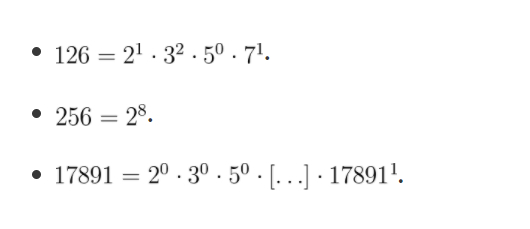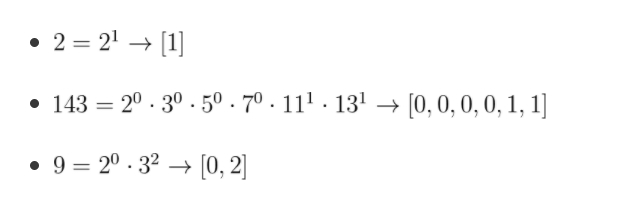# 脑洞：如何用一个整数来表示一个列表？Python猫

## 概要

>>> import math>>> math.factorial(2020)[number omitted]  # Python猫注：此处求2020的阶乘，结果是一长串数字，所以省略>>> math.log2(math.factorial(2020))19272.453841606068>>> type(math.factorial(2020))<class 'int'>

*(Python 猫注：这有一篇文章 ，深度剖析了 Python 整型不会溢出的实现原理，可作关联阅读)*

## 哥德尔数（Gödel numbering）简介

（Python 猫注：质数分解，即 prime factorization，又译作质因数分解、素因子分解等，指的是把每个数都写成用质数相乘的形式）## Python 实现

1. 我们会使用带有 yield 的函数，因为它极大地简化了操作。[[注 5]](https://iantayler.com/2020/12/07/storing-a-list-in-an-int/%23footnotes#footnotes)

2. 你会看到大量的 while 循环。这是因为列表生成式、range 和大多数你打算在 for 循环中使用的东西，都被禁止用在只有 int 类型的方言中。所有这些都被 while 循环替代了。

### 质数生成器

def primes(starting: int = 2):    """Yield the primes in order.         Args:        starting: sets the minimum number to consider.         Note: starting can be used to get all prime numbers    _larger_ than some number. By default it doesn't skip    any candidate primes.    """    candidate_prime = starting    while True:        candidate_factor = 2        is_prime = True        # We'll try all the numbers between 2 and        # candidate_prime / 2. If any of them divide        # our candidate_prime, then it's not a prime!        while candidate_factor <= candidate_prime // 2:            if candidate_prime % candidate_factor == 0:                is_prime = False                break            candidate_factor += 1        if is_prime:            yield candidate_prime        candidate_prime += 1

### 创建空列表

def empty_list() -> int:    """Create a new empty list."""    # 1 is the empty list. It isn't divisible by any prime.    return 1

### 遍历元素

def iter_list(l: int):    """Yields elements in the list, from first to last."""    # We go through each prime in order. The next value of    # the list is equal to the number of times the list is    # divisible by the prime.    for p in primes():        # We decided we will have no trailing 0s, so when        # the list is 1, it's over.        if l <= 1:            break        # Count the number of divisions until the list is        # not divisible by the prime number.        num_divisions = 0        while l % p == 0:            num_divisions += 1            l = l // p  # could be / as well        yield num_divisions

### 访问元素

def access(l: int, i: int) -> int:    """Return i-th element of l."""    # First we iterate over all primes until we get to the    # ith prime.    j = 0    for p in primes():        if j == i:            ith_prime = p            break        j += 1    # Now we divide the list by the ith-prime until we    # cant divide it no more.    num_divisions = 0    while l % ith_prime == 0:        num_divisions += 1        l = l // ith_prime    return num_divisions

### 添加元素

def append(l: int, elem: int) -> int:    # The first step is finding the largest prime factor.    # We look at all primes until l.    # The next prime after the last prime factor is going    # to be the base we need to use to append.    # E.g. if the list if 18 -> 2**1 * 3**2 -> [1, 2]    # then the largest prime factor is 3, and we will    # multiply by the _next_ prime factor to some power to    # append to the list.    last_prime_factor = 1  # Just a placeholder    for p in primes():        if p > l:            break        if l % p == 0:            last_prime_factor = p    # Now get the _next_ prime after the last in the list.    for p in primes(starting=last_prime_factor + 1):        next_prime = p        break    # Now finally we append an item by multiplying the list    # by the next prime to the elem power.    return l * next_prime ** elem

### 试用这些函数

In : l = empty_list() In : l = append(l, 2) In : l = append(l, 5) In : list(iter_list(l))Out: [2, 5] In : access(l, 0)Out: 2 In : access(l, 1)Out: 5 In : lOut: 972  # Python猫注：2^2*3^5=972

## 脚注

1. 我认为在内存不足之前，程序也会出现中断，但是文档确实明确地提到它们具有无限的精度

2. 请注意，对于 Python3，这是正确的，但对于 Python2 则不然。对于 Python2，int 是固定大小的。我认为在 2020 年用 Python 指代 Python3 是没问题的，但我也认为这个细节值得加一条脚注。

3. 对于用哥德尔数表示列表，这很容易被反驳说是一种糟糕的表示形式。在后续的博文中，我们会讨论有关表示形式的权衡问题。

4. 我们可以将列表的长度存储在单独的 int 中，据此知道要在列表末尾考虑多少个 0。（猫注：还有几句话没看懂，不译）If we don’t want to have a whole separate int, we can always write the length of the list as the exponent of 2 and start the actual list with the exponent of 3. This has some redundant information, though. The way to avoid redundant information is to store the number of final 0s in the list, instead of the entire length. We won’t be worrying about any of this, though.

5. 请注意，跟使用 return 并将状态变量作为参数相比，使用 yield 没有区别（通常足以获得最后一个返回的元素）。这有点像 Continuation Passing Style。也类似于平常的使非尾递归函数尾递归的累加器。如果你从未听说过累加器技巧，这里有一些链接[](https://raganwald.com/2018/05/27/tail.html) 、[](https://blog.appsignal.com/2019/03/19/elixir-alchemy-recursion.html) 。我未来可能会在没有它们的语言中，写模仿迭代器的东西。

6. 另请参见《 The Genuine Sieve of Erathosthenes》论文，它澄清了这一算法是如何被定义的。

Python 猫注： 以上是全部译文，但我最后还想补充一个有趣的内容。在《黑客与画家》中，保罗·格雷大师有一个惊人的预言，他认为在逻辑上不需要有整数类型，因为整数 n 可以用一个 n 元素的列表来表示。哈哈，这跟上文的脑洞恰好反过来了！想象一下，一个只有整数类型没有列表的编程语言，以及一个只有列表类型没有整数的编程语言，哪一个更有可能在未来出现呢？### Python猫

Python猫作者，一个Python爱好者 2018.08.07 加入

## 评论 (1 条评论)